# Introduction

In a location problem, we seek to find an optimal location of a service, or a set of them, so that the quality that the service provides to a set of demand points is, according to a given performance measure, optimal.

Some examples of localization problems are:

• Find the optimal location of the central warehouse of a merchandise distribution network so that the total cost of transport is minimized
• Find the optimal location of an ambulance that must assist to the patients of a certain region so that the time in treating the farthest patient is minimized

There are numerous contexts in which location problems arise, due to this, the location theory has been object of great attention in recent years, being able to say that it is a subject of great importance. The appearance of new facets of the problem, didn’t handle until now, contributes to this. For example, along with the already classic criteria for minimizing costs, new criterias appear: environmental, social, quality of life, etc. These new aspects of the problem make it an open field of study.

The package presented is devoted to solving the problem of locating a single point in the plane, using as an objective the minimization of the sum of the weighted distances to the demand points. New versions of the package will include new location models.

The package RcmdrPlugin.UCA adds a set of options to the Rcmdr menu to solve planar location problems. The main entry for this menu is Orloca, and the options in this menu are:

• New loca.p
• New loca.p Random Instance
• Summary
• Evaluation of Objective Function for weighted sum Location Problem
• Solve weighted sum Location Problem
• Graphics
• Options
• Help

# The class of objects loca.p

In a planar location problem the set of demand points is given by the coordinates of said points. Optionally, a set of weights can be assigned to said points, which gives more importance to some points than to others, since the objective considered is to minimize the weighted sum of the distances between the service point and said requesting assembly. For example, if the location of a regional hospital is sought, the demand points can be the localities to which the hospital must attend and the weights of the population of each locality.

For the resolution of these problems, a class of objects designated loca.p has been defined, so that a loca.p object stores the coordinates of the demand points and the weights of each of the points. Each object loca.p has three slots, x and y that store the coordinates and w that stores the weights. When the weights are not given explicitly, all the points of demand will be considered equally important.

The rest of this section will explain how to do basic operations with loca.p objects.

## Creating class objects loca.p

Consider a localization problem in which the set of demand points is $$(0,0)$$, $$(4,0)$$ and $$(2,2)$$. To create a loca.p object that represents that set, the options “Orloca” -> “New loca.p” are selected in the menu, obtaining the dialog:Dialog “New loca.p”

Changing the name and clicking on “OK” accesses the data editor window. In the x column the values $$0$$, $$4$$, $$2$$ and $$0$$, $$0$$, $$2$$ are entered in the y column, and finally, three ones are entered in the w column. When leaving the editor, a new data set loca1 representing a loca.p object has been created and activated.

The necessary conversions between the data.frame type and the loca.p type are done automatically.

## Random generation of class objects loca.p

You can create random objects of class loca.p using the options “Orloca” -> “New loca.p Random Instance”, obtaining the dialog: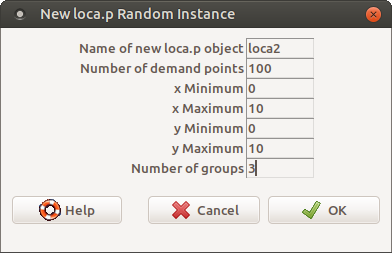Dialog “New loca.p Random Instance”

Changing the dialog fields as shown in the figure, a new object loca.p of name loca2 is generated with 100 demand points with both coordinates between 0 and 100 and with three groups. After clicking on OK in the instructions window you get:

loca2 <- rloca.p(n = 100, xmin = 0, xmax = 10, ymin = 0, ymax = 10, groups = 3)
loca2 <- as(loca2, "data.frame")

The new active data set becomes loca2 and can be viewed using the “Display data set” button.

## Summarizing the data

A numeric summary of a loca.p object can be obtained. The summary shows the minimum, maximum and average values of both coordinates, in addition to the weighted averages of the coordinates of the points for each component.

By previously activating the data set loca1 and selecting “Orloca” -> “Summary”, you get:

summary(loca1)
#>        x           y                w
#>  Min.   :0   Min.   :0.0000   Min.   :1
#>  1st Qu.:1   1st Qu.:0.0000   1st Qu.:1
#>  Median :2   Median :0.0000   Median :1
#>  Mean   :2   Mean   :0.6667   Mean   :1
#>  3rd Qu.:3   3rd Qu.:1.0000   3rd Qu.:1
#>  Max.   :4   Max.   :2.0000   Max.   :1

# Weighted average distance

Given a loca.p object, we can evaluate the weighted average distance from a given point. Likewise, the gradient of said function can be evaluated and the problem of minimizing said objective can be solved.

## Evaluation

The weighted average distance function is called distsum in the package. Given a point, for example: $$(3, 1)$$ to evaluate the weighted average distance to loca1, chose from the menu “Orloca” -> “Evaluation of the Objective Function of the weighted sum Location Problem” and the dialog is obtained:Dialog “Evaluation of the Objective Function of the weighted sum Location Problem”

By entering the values shown you get:

distsum(as(loca1, "loca.p"), x = 3, y = 1) # Weighted sum of the distances
#>  5.990705
distsumgra(as(loca1, "loca.p"), x = 3, y = 1) # Gradient of the weighted sum function of the distances
#>  0.9486833 0.3162278

Note that the gradient at that point of the objective function has also been obtained.

## Resolution

To find the optimal solution to the previous location problem, select “Orloca” -> “Solve weighted sum Location Problem”, obtaining the dialog box: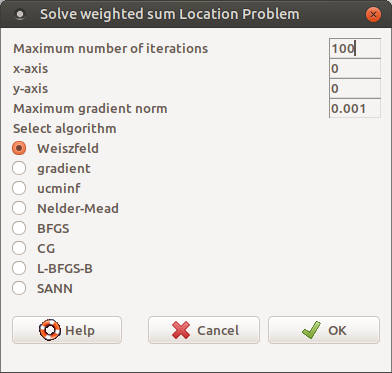Dialog “Solve weighted sum Location Problem”

And clicking on “OK” you get:

.sol <- distsummin(as(loca1, "loca.p"), x = 0, y = 0, eps = 0.001, algorithm = "Weiszfeld") # Solve the location problem minsum
.sol # Show the solution
#>  2.00000 1.15332
distsum(as(loca1, "loca.p"), x = 2.00000022259505, y = 1.15332010901434) # Weighted sum of the distances
#>  5.464102
remove(.sol)

Where it is shown that the solution is the point $$(2, 1.15)$$, the function is evaluated in it, resulting in a value of $$5.46$$ and a virtually zero gradient, which indicates that the point is an extreme point. The solution found is a local optimum and since the convex objective function is a global optimum.

# Plotting

Both the objects loca.p and the objective function can be represented in a graph. For the objective function, a representation based on level curves and another one on a 3D graph is provided.

## Plotting an object loca.p

The graph of a loca.p object consists of plotting the dispersion diagram on the plane of the set of demand points. Choosing “Orloca” -> “Graphs” -> “Graph of the set of demand points”, you get:

plot(as(loca1, "loca.p"), main = "Graph of the set of points loca1")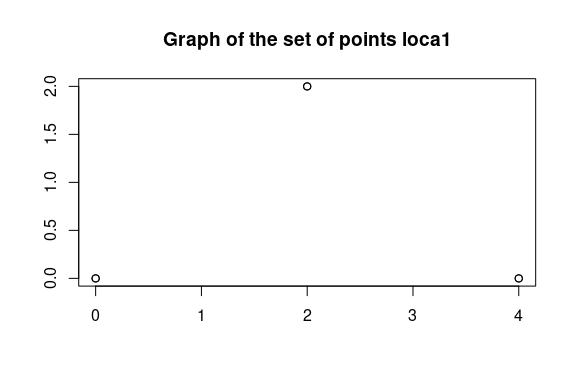## Graph of contour lines

The graph of contours is done by choosing “Orloca” -> “Graphs” -> “Graph of contours of distsum”, obtaining:

contour(as(loca1, "loca.p"), main = "Graph of the contours of the objective function for loca1")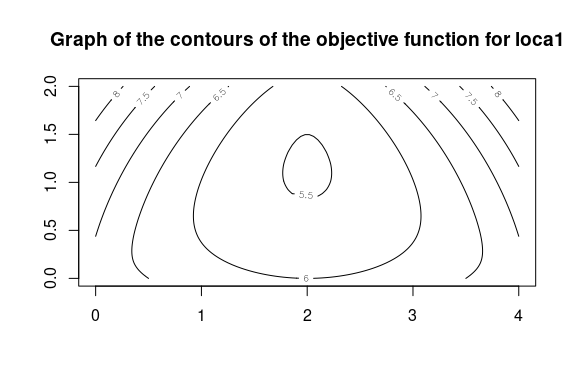In the graph you can see how the function reaches the minimum at the point previously calculated. Selecting “Orloca” -> “Graphs” -> “Demand graphs and contour lines” you get the two previous graphs superimposed:

plot(as(loca1, "loca.p"), main = "Demand points and level curves for loca1")
contour(as(loca1, "loca.p"), add = T)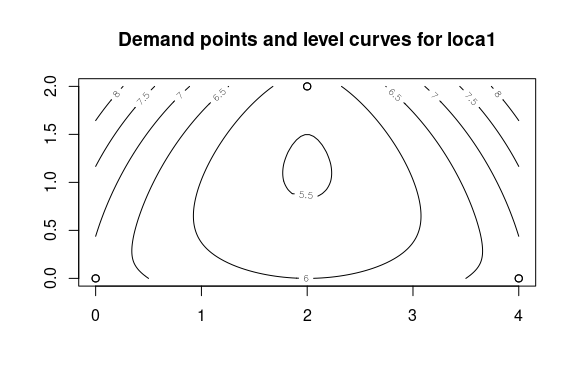## Graph in 3D

A three-dimensional representation can be made by selecting the options “Orloca” -> “Graphics” -> “3D graph of distsum”, obtaining:

persp(as(loca1, "loca.p"), main = "3D graph of the objective function min-sum for loca1")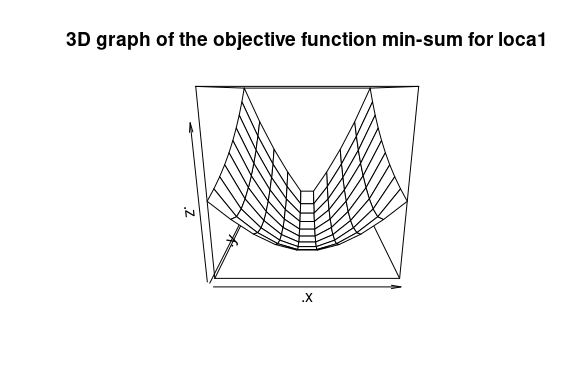# Use of other norm

The norm that is used by default is the Euclidean norm or $$l_2$$ norm. Through the options “Orloca” -> “Options” -> “Show/Set norm” you can display the norm in use or choose a new norm within the $$l_p$$ family of norms.

When choosing those options from the menu, we get the dialog: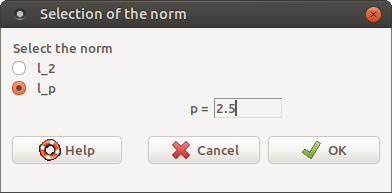Dialog “Show/Set norm”

In this dialog you can specify a new value for $$p$$ greater than or equal to 1, and that otherwise, $$l_p$$ would not be a norm. All calculations and graphs will be made using this norm until a new value is specified or the norm $$l_2$$ is chosen again.

The new contour graph is:

contour(as(loca1, "loca.p"), main = "Graph of the level curves of the objective function for loca1 \n (Standard lp = 2.5)", lp = 2.5)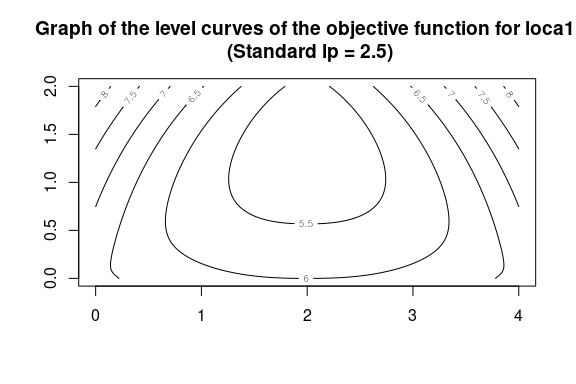The presented package allows solving the problem of planar location of a single service with norm $$l_p$$ using an user friendly menus.